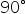Maths-
General
Easy

Question

# if the angle is less than 90. Than it is called _______ angle:

## The correct answer is: Acute angle

### If the angle is less thanthen it is called acute angle.If the angle is more thanthen it is called obtuse angle.If the angle is equal tothen it is called Right angle.#### With Turito Foundation.#### Get an Expert Advice From Turito.